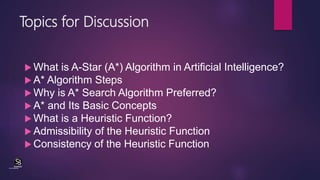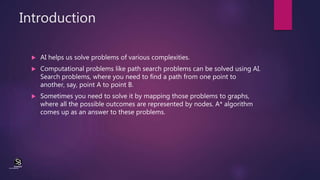Ce diaporama a bien été signalé.
Le téléchargement de votre SlideShare est en cours. ×
Publicité
Publicité
Publicité
Publicité
Publicité
Publicité
Publicité
Publicité
Publicité
Publicité
PublicitéChargement dans…3
×

## Consultez-les par la suite

1 sur 15 Publicité

# A* Algorithm

This presentation discuses the following topics:
What is A-Star (A*) Algorithm in Artificial Intelligence?
A* Algorithm Steps
Why is A* Search Algorithm Preferred?
A* and Its Basic Concepts
What is a Heuristic Function?
Consistency of the Heuristic Function

This presentation discuses the following topics:
What is A-Star (A*) Algorithm in Artificial Intelligence?
A* Algorithm Steps
Why is A* Search Algorithm Preferred?
A* and Its Basic Concepts
What is a Heuristic Function?
Consistency of the Heuristic Function

Publicité
Publicité

### A* Algorithm

1. 1. A* Algorithm Dr. C.V. Suresh Babu (CentreforKnowledgeTransfer) institute
2. 2. Topics for Discussion  What is A-Star (A*) Algorithm in Artificial Intelligence?  A* Algorithm Steps  Why is A* Search Algorithm Preferred?  A* and Its Basic Concepts  What is a Heuristic Function?  Admissibility of the Heuristic Function  Consistency of the Heuristic Function (CentreforKnowledgeTransfer) institute
3. 3. Introduction  AI helps us solve problems of various complexities.  Computational problems like path search problems can be solved using AI. Search problems, where you need to find a path from one point to another, say, point A to point B.  Sometimes you need to solve it by mapping those problems to graphs, where all the possible outcomes are represented by nodes. A* algorithm comes up as an answer to these problems. (CentreforKnowledgeTransfer) institute
4. 4.  Created as part of the Shakey project aimed to build a mobile robot that has artificial intelligence to plan its actions, A* was initially designed as a general graph traversal algorithm.  It is widely used in solving pathfinding problems in video games. Because of its flexibility and versatility, it can be used in a wide range of contexts.  A* is formulated with weighted graphs, which means it can find the best path involving the smallest cost in terms of distance and time.  This makes A* algorithm in artificial intelligence an informed search algorithm for best-first search.  Let us have a detailed look into the various aspects of A*. (CentreforKnowledgeTransfer) institute
5. 5. What is A* Algorithm in Artificial Intelligence?  The most important advantage of A* search algorithm which separates it from other traversal techniques is that it has a brain.  This makes A* very smart and pushes it much ahead of other conventional algorithms. (CentreforKnowledgeTransfer) institute
6. 6.  Let’s try to understand Basic AI Concepts and to comprehend how does A* algorithm work.  Imagine a huge maze, one that is too big that it takes hours to reach the endpoint manually.  Once you complete it on foot, you need to go for another one.  Which implies that you would end up investing a lot of time and effort to find the possible paths in this maze.  Now, you want to make it less time-consuming.  To make it easier, we will consider this maze as a search problem and will try to apply it to other possible mazes we might encounter in the due course, provided they follow the same structure and rules. (CentreforKnowledgeTransfer) institute
7. 7. Converting Maze into a search problem As the first step to convert this maze into a search problem, we need to define these six things. 1. A set of prospective states we might be in 2. A beginning and end state 3. A way to decide if we’ve reached the endpoint 4. A set of actions in case of possible direction/path changes 5. A function that advises us about the result of an action 6. A set of costs incurring in different states/paths of movement (CentreforKnowledgeTransfer) institute
8. 8.  To solve the problem, we need to map the intersections to the nodes (denoted by the red dots) and all the possible ways we can make movements towards the edges (denoted by the blue lines).  A denotes the starting point and B denotes the endpoint. We define the starting and endpoint at the nodes A and B respectively.  If we use an uninformed search algorithm, it would be like finding a path that is blind, while an informed algorithm for a search problem would take the path that brings you closer to your destination. For instance, consider Rubik’s cube; it has many prospective states that you can be in and this makes the solution very difficult. This calls for the use of a guided search algorithm to find a solution. This explains the importance of A*. (CentreforKnowledgeTransfer) institute
9. 9. Why A*?  Unlike other algorithms, A* decides to take up a step only if it is convincingly sensible and reasonable as per its functions.  Which means, it never considers any non-optimal steps.  This is why A* is a popular choice for AI systems that replicate the real world – like video games and machine learning. (CentreforKnowledgeTransfer) institute
10. 10. A* Algorithm Steps  Firstly, add the beginning node to the open list  Then repeat the following step  – In the open list, find the square with the lowest F cost – and this denotes the current square. – Now we move to the closed square. – Consider 8 squares adjacent to the current square and  Ignore it if it is on the closed list, or if it is not workable. Do the following if it is workable  Check if it is on the open list; if not, add it. You need to make the current square as this square’s a parent. You will now record the different costs of the square like the F, G and H costs.  If it is on the open list, use G cost to measure the better path. Lower the G cost, the better the path. If this path is better, make the current square as the parent square. Now you need to recalculate the other scores – the G and F scores of this square.  – You’ll stop:  If you find the path, you need to check the closed list and add the target square to it.  There is no path if the open list is empty and you could not find the target square.  3. Now you can save the path and work backwards starting from the target square, going to the parent square from each square you go, till it takes you to the starting square. You’ve found your path now. (CentreforKnowledgeTransfer) institute
11. 11. Why is A* Search Algorithm Preferred?  The task is to take the unit you see at the bottom of the diagram, to the top of it. You can see that there is nothing to indicate that the object should not take the path denoted with pink lines.  So it chooses to move that way. As and when it reaches the top it has to change its direction because of the ‘U’ shaped obstacle.  Then it changes the direction, goes around the obstacle, to reach the top. In contrast to this, A* would have scanned the area above the object and found a short path (denoted with blue lines).  Thus, pathfinder algorithms like A* help you plan things rather than waiting until you discover the problem.  They act proactively rather than reacting to a situation.  The disadvantage is that it is a bit slower than the other algorithms.  You can use a combination of both to achieve better results – pathfinding algorithms give bigger picture and long paths with obstacles that change slowly; and movement algorithms for local picture and short paths with obstacles that change faster. It’s easy to give movement to objects. But pathfinding is not simple. It is a complex exercise. The following situation explains it. (CentreforKnowledgeTransfer) institute
12. 12. A* Algorithm and Its Basic Concepts  A* algorithm works based on heuristic methods and this helps achieve optimality.  A* is a different form of the best-first algorithm.  Optimality empowers an algorithm to find the best possible solution to a problem.  Such algorithms also offer completeness, if there is any solution possible to an existing problem, the algorithm will definitely find it. When A* enters into a problem, firstly it calculates the cost to travel to the neighbouring nodes and chooses the node with the lowest cost.  If The f(n) denotes the cost, A* chooses the node with the lowest f(n) value.  Here ‘n’ denotes the neighbouring nodes. The calculation of the value can be done as shown below: f(n)=g(n)+h(n)f(n)=g(n)+h(n) g(n) = shows the shortest path’s value from the starting node to node n h(n) = The heuristic approximation of the value of the node  The heuristic value has an important role in the efficiency of the A* algorithm.  To find the best solution, you might have to use different heuristic function according to the type of the problem.  However, the creation of these functions is a difficult task, and this is the basic problem we face in AI. (CentreforKnowledgeTransfer) institute
13. 13. What is a Heuristic Function?  A heuristic as it is simply called, a heuristic function that helps rank the alternatives given in a search algorithm at each of its steps.  It can either produce a result on its own or work in conjugation with a given algorithm to create a result.  Essentially, a heuristic function helps algorithms to make the best decision faster and more efficiently.  This ranking is made based on the best available information and helps the algorithm to decide on the best possible branch to follow.  Admissibility and consistency are the two fundamental properties of a heuristic function. (CentreforKnowledgeTransfer) institute
14. 14. Admissibility of the Heuristic Function  A heuristic function is admissible if it could effectively estimate the real distance between a node ‘n’ and the end node.  It never overestimates and if it ever does, it will be denoted by ‘d’, which also denotes the accuracy of the solution. (CentreforKnowledgeTransfer) institute
15. 15. Consistency of the Heuristic Function  A heuristic function is consistent if the estimate of a given heuristic function turns out to be equal to, or less than the distance between the goal (n) and a neighbour, and the cost calculated to reach that neighbour.  A* is indeed a very powerful algorithm used to increase the performance of artificial intelligence. It is one of the most popular search algorithms in AI.  Sky is the limit when it comes to the potential of this algorithm. However, the efficiency of an A* algorithm highly depends on the quality of its heuristic function.  Wonder why this algorithm is preferred and used in many software systems?  There is no single facet of AI where A*algorithm has not found its application. From search optimization to games, robotics and machine learning, A* algorithm is an inevitable part of a smart program. (CentreforKnowledgeTransfer) institute